# How to deal with Floating-Point Rounding Error

So, in our day to day programing we frequently deal with floating point numbers. But the thing is when you do calculations with floating point numbers you have to aware about certain things. If you have no idea about fundamentals and how computer deals with the floating-point numbers, you may have to deal with some hard situations that occurs by these even without your knowledge.

Now I will show you really simple calculation using floating-point numbers.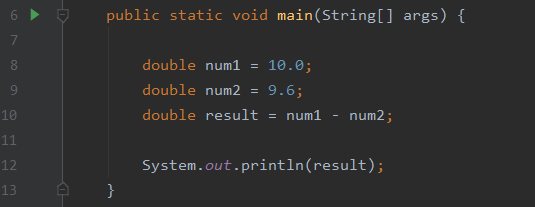Image: Calculations using Floating-Point numbers 01

As you can see it is a simple calculation and you obviously have the answer right now. So, the correct answer should be 0.4. But here is the answer that provide by our machine.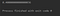Image: Answer 01

Not the exact answer as you calculated right? There is a reason for that, computer face to a problem when it has to use floating-point numbers. That is called as “Floating-Point Rounding Error”. Before moving into the Floating-Point Rounding Error, lets understand fundamentals about Floating-Point numbers.

## IEEE 754 Standard

In computer there is a standard called IEEE 754 that used to represent the floating-points numbers. IEEE 754 has 3 main components named as Sign, Exponent, and Mantissa.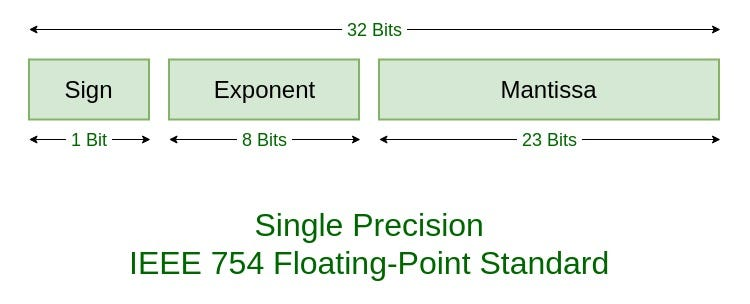Image: IEEE 754 Standard for Single Precision (By: www.geeksforgeeks.org)
• Sign: This is basically representing the sign of the number (Positive or Negative).
• Exponent: This field represents both positive and negative exponents. A bias is added to the actual exponent in order to get the stored exponent.
• Mantissa: This is part of a number in scientific notation or a floating-point number, consisting of its significant digits.

In the following image you will see how these 3 components represents according to the Single precision, Double Precision, and Long Double Precision.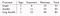Image: Single, Double, Long-Double precision’s representation

Let’s clarify this using an example. In this example I will use single precision format and “9.1” as the floating-point number.

i) At first, we have to convert 9.1 into binary.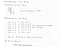Image: Converting 9.1 to binary

Result of converting 9.1 into binary: 1001.0001100110011001100…

ii) Now we have to write this in a Scientific Notation. In the scientific notation the binary number look like this,

1.0010001100110011001100… * 23

iii) Now we have to convert this into IEEE 754 standard. Remember in this example we are using “single precision format”.

• In IEEE standard the first bit represents the sign. So, if the sign positive the value will be “0”, and if the sign is negative the value will be “1”. In our case it is positive, that means value will be 0.
• Now we have to consider the Exponent. In this scenario our exponent value is 2^3 (2 to the power 3). So, the Exponent has value of 8 bits to represent itself and exponent should represent both positive and negative (-128 to 127). Before moving to the last part, there is another thing to consider. That is if our exponent is positive, we must add that into the 8-bit representation. So, our exponent is 3 (2^3) and we must add it into the 127, then it becomes 130. Finally convert 130 into binary and it will represent the “Exponent value” in IEEE 754 standard.

130 into binary -> 10000010

• Now all we left to do is add the 9.1’s Binary Scientific Notation as the Mantissa. So, after all these calculations, 9.1’s IEEE 754 representation is look like this.

01000001000100011001100110011001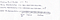Image: IEEE 754 Representation of 9.1

So, this is how all the modern-day machines handle Floating-Points internally. But the thing is if you try to convert 9.1 into IEEE 754 representation using a computer, it won’t give the exact answer as above.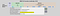Image: Binary Representation of 9.1 by using computer

To access IEEE-754 Floating Point Converter: https://www.h-schmidt.net/FloatConverter/IEEE754.html

So, this is the so-called Floating-Point Rounding Error. Lets see why this Floating-Point Rounding Error occur and what we can do prevent it.

# Why Floating-Point Rounding Error occur in computer?

As we all know computers don’t understand letters and numbers as we do. So, it converts everything into binary to understand. Same goes with floating-point numbers as well. Computer converts floating-point numbers to understand. But the thing is when computer converts those binaries back again into the floating-point number it won’t give the exact result.

If you read the article well you already know that there are 3 formats given by IEEE to represent floating-point numbers in computer. So, in single precision it has total 32 bits to represent a floating-point number. When computer convert a floating-point number into binary, Mantissa have to hold that binary value (Binary Scientific Notation). But Mantissa can only represent up to 23 digits. However, what computer does is, it going to round from that last digit Mantissa can hold. So that’s why computer won’t give us the exact result when using floating-point numbers. This is what we called as Floating-Point Rounding Error.

However, we can prevent this from happening by using appropriate data-types such as, “BigDecimal Class” or we can still use “Float and Double” by limiting their decimals points.

# Using BigDecimal Class

If you want to use floating-point numbers to do calculations and get the exact results, then you can use BigDecimal class in Java.

The BigDecimal class provides operations on floating-point numbers for arithmetic, comparison, rounding, and hashing. It can handle very large and very small floating-point numbers with great precision.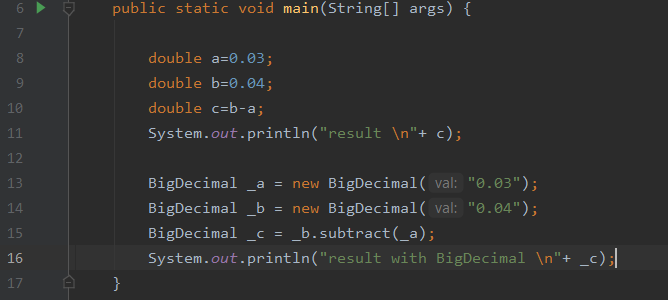Image: Calculations using Floating-Point numbers 02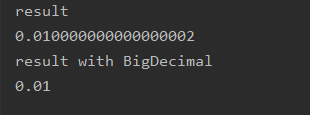Image: Answer 02

As you can see, using BigDecimal class we can do calculations on floating-point numbers and get the exact results without facing to Floating-Point Rounding Error. Also, there are various methods that you can use to do arithmetic operations using BigDecimal class.Image: Methods from BigDecimal Class

## References

How Computer deal with Floating point numbers | Decimal to IEEE 754 Floating point Representation. 2020. [video] Directed by K. Dinesh. https://www.youtube.com/watch?v=2VM028vpguU&t=7s: YouTube.

GeeksforGeeks. 2020. IEEE Standard 754 Floating Point Numbers — GeeksforGeeks. [online] Available at: <https://www.geeksforgeeks.org/ieee-standard-754-floating-point-numbers/> [Accessed 6 May 2021].

GeeksforGeeks. 2018. BigDecimal Class in Java — GeeksforGeeks. [online] Available at: <https://www.geeksforgeeks.org/bigdecimal-class-java/> [Accessed 7 May 2021].

Associate Software Engineer at Virtusa

## More from Nisal Pubudu

Associate Software Engineer at Virtusa